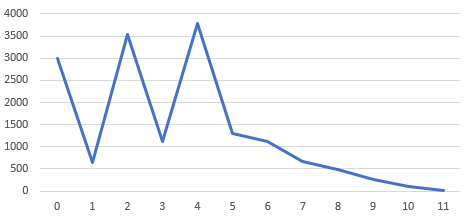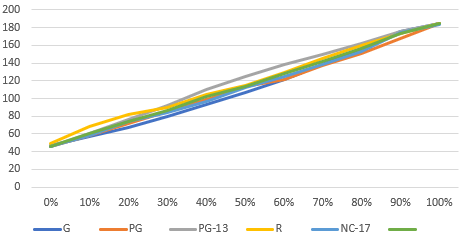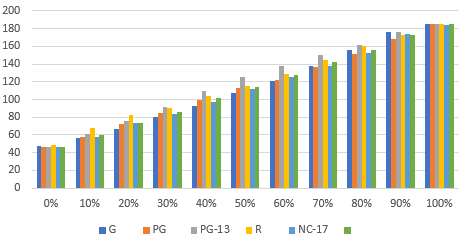## Calculate Percentiles to Learn About Data Set Skew in SQL

B-Tree indexes are perfect when your data is uniformly distributed. They are not really useful, when you have skewed data. I’ll explain later why this is the case, but let’s first learn how to detect “skew”

### What is skew?

Skew is a term from statistics when a normal distribution is not symmetric. The example given on Wikipedia shows a distribution like this:In RDBMS, we sometimes use the term skew colloquially to mean the same thing as non-uniform distribution, i.e. a normal distribution would also be skewed. We simply mean that some values appear more often than others. Thus, I will put the term “skew” in double quotes in this article. While your RDBMS’s statistics contain this information once they are calculated, we can also detect such “skew” manually in ad-hoc queries using percentiles, which are defined in the SQL standard and supported in a variety of databases, as ordinary aggregate functions, including:
• Oracle
• PostgreSQL
• SQL Server (regrettably, only as window functions)
Uniform distribution Let’s look at the FILM_ID values in the Sakila database:
```
SELECT
percentile_disc(0.0) WITHIN GROUP (ORDER BY film_id) AS "0%",
percentile_disc(0.1) WITHIN GROUP (ORDER BY film_id) AS "10%",
percentile_disc(0.2) WITHIN GROUP (ORDER BY film_id) AS "20%",
percentile_disc(0.3) WITHIN GROUP (ORDER BY film_id) AS "30%",
percentile_disc(0.4) WITHIN GROUP (ORDER BY film_id) AS "40%",
percentile_disc(0.5) WITHIN GROUP (ORDER BY film_id) AS "50%",
percentile_disc(0.6) WITHIN GROUP (ORDER BY film_id) AS "60%",
percentile_disc(0.7) WITHIN GROUP (ORDER BY film_id) AS "70%",
percentile_disc(0.8) WITHIN GROUP (ORDER BY film_id) AS "80%",
percentile_disc(0.9) WITHIN GROUP (ORDER BY film_id) AS "90%",
percentile_disc(1.0) WITHIN GROUP (ORDER BY film_id) AS "100%"
FROM film;

```
What are we calculating here? We’re trying to find 11 different values for which we can say that:
• 0% of the film_ids are lower than the “0%” value
• 10% of the film_ids are lower than the “10%” value
Or in other words:
• 0% is the MIN(film_id) value
• 50% is the MEDIAN(film_id) value
• 100% is the MAX(film_id) value
The result shows an unsurprisingly uniform distribution:
```0% |10% |20% |30% |40% |50% |60% |70% |80% |90% |100% |
---|----|----|----|----|----|----|----|----|----|-----|
1  |100 |200 |300 |400 |500 |600 |700 |800 |900 |1000 |
```
We can plot this in Microsoft Excel or some other tool to get this nice curve:This is not surprising, as the IDs are just consecutive values, which is a desired property of surrogate keys. “Skewed” distribution It’s a different story when we look at the distribution of amounts in the payment table:
```
SELECT
percentile_disc(0.0) WITHIN GROUP (ORDER BY amount) AS "0%",
percentile_disc(0.1) WITHIN GROUP (ORDER BY amount) AS "10%",
percentile_disc(0.2) WITHIN GROUP (ORDER BY amount) AS "20%",
percentile_disc(0.3) WITHIN GROUP (ORDER BY amount) AS "30%",
percentile_disc(0.4) WITHIN GROUP (ORDER BY amount) AS "40%",
percentile_disc(0.5) WITHIN GROUP (ORDER BY amount) AS "50%",
percentile_disc(0.6) WITHIN GROUP (ORDER BY amount) AS "60%",
percentile_disc(0.7) WITHIN GROUP (ORDER BY amount) AS "70%",
percentile_disc(0.8) WITHIN GROUP (ORDER BY amount) AS "80%",
percentile_disc(0.9) WITHIN GROUP (ORDER BY amount) AS "90%",
percentile_disc(1.0) WITHIN GROUP (ORDER BY amount) AS "100%"
FROM payment;

```
We’re now getting:
```0%   |10%  |20%  |30%  |40%  |50%  |60%  |70%  |80%  |90%  |100%
-----|-----|-----|-----|-----|-----|-----|-----|-----|-----|-----
0.00 |0.99 |1.99 |2.99 |2.99 |3.99 |4.99 |4.99 |5.99 |6.99 |11.99
```
This looks … “skewed”, although clearly the bias is mainly caused by the fact that this data is generated. When we plot the above, we’re getting:The slope is less steep at the beginning of this curve, which essentially means that more values exist at the lower end of the range than at the upper end. We can validate this with another query:
```
SELECT amount, count(*)
FROM (
SELECT trunc(amount) AS amount
FROM payment
) t
GROUP BY amount
ORDER BY amount;

```
… which yields:
```amount |count |
-------|------|
0      |3003  |
1      |641   |
2      |3542  |
3      |1117  |
4      |3789  |
5      |1306  |
6      |1119  |
7      |675   |
8      |486   |
9      |257   |
10     |104   |
11     |10    |
```
Plotted:When plotting this, we can see that there are more amounts in the lower half of the range than in the upper half, which leads to percentiles growing slower. Correlations This technique can also be applied to detect correlations in data. We can, for instance, try to find the percentiles of the length of films, and group data sets by rating. I’m using a GROUPING SETS function here, the ROLLUP() function, to calculate the grand total as well. Just check out the query and its results, and you’ll see:
```
SELECT
rating,
count(*),
percentile_disc(0.0) WITHIN GROUP (ORDER BY length) AS "0%",
percentile_disc(0.1) WITHIN GROUP (ORDER BY length) AS "10%",
percentile_disc(0.2) WITHIN GROUP (ORDER BY length) AS "20%",
percentile_disc(0.3) WITHIN GROUP (ORDER BY length) AS "30%",
percentile_disc(0.4) WITHIN GROUP (ORDER BY length) AS "40%",
percentile_disc(0.5) WITHIN GROUP (ORDER BY length) AS "50%",
percentile_disc(0.6) WITHIN GROUP (ORDER BY length) AS "60%",
percentile_disc(0.7) WITHIN GROUP (ORDER BY length) AS "70%",
percentile_disc(0.8) WITHIN GROUP (ORDER BY length) AS "80%",
percentile_disc(0.9) WITHIN GROUP (ORDER BY length) AS "90%",
percentile_disc(1.0) WITHIN GROUP (ORDER BY length) AS "100%"
FROM film
GROUP BY ROLLUP(rating);

```
This yields:
```rating |count |0% |10% |20% |30% |40% |50% |60% |70% |80% |90% |100% |
-------|------|---|----|----|----|----|----|----|----|----|----|-----|
G      |178   |47 |57  |67  |80  |93  |107 |121 |138 |156 |176 |185  |
PG     |194   |46 |58  |72  |85  |99  |113 |122 |137 |151 |168 |185  |
PG-13  |223   |46 |61  |76  |92  |110 |125 |138 |150 |162 |176 |185  |
R      |195   |49 |68  |82  |90  |104 |115 |129 |145 |160 |173 |185  |
NC-17  |210   |46 |58  |74  |84  |97  |112 |125 |138 |153 |174 |184  |
|1000  |46 |60  |74  |86  |102 |114 |128 |142 |156 |173 |185  |
```
So, the `GROUP BY` clause produced one row per rating, and an additional grand total column at the bottom. For illustration purposes, I’ve added the `COUNT(*)` column, to show how many films are in each group. The 5 first rows sum up to 1000, which is again the grand total at the bottom. Let’s plot the percentiles now as line and bar charts:We can “see” that there is no strong correlation between the two data points. Both data sets are close to uniformly distributed, quite independently of the rating, with the exception of PG-13, which is just slightly skewed towards longer film lengths. Again, this isn’t terribly interesting as the data set was generated, probably using some randomness to avoid perfectly uniform distribution. In real world scenarios, the above data would have been more “skewed”.

### How does this help with performance?

A balanced tree index is very useful when data is quite uniformly distributed, because in that case, it can help access data points or ranges of data in O(log(N)) time. This is quite a useful property for queries that look for film_id values, e.g.
```
SELECT *
FROM film
WHERE film_id = 1

```
When accessing “skewed” data, some values are more equal than others. This means that for example if we’re looking for amounts in the payment table, these two queries are not the same:
```
-- A lot of rows returned (3644)
SELECT * FROM payment WHERE amount BETWEEN 0 AND 2;

-- Few rows returned (361)
SELECT * FROM payment WHERE amount BETWEEN 9 AND 11;

```
An index on the amount column could have been useful for the second query, but maybe not for the first one. There are several things we can do to make sure optimal index usage is being applied for all sorts of queries. In case of uniformly distributed data, we usually don’t have to do anything as SQL developers. In case of “skewed” data sets, it may be worth thinking about:
• Using histogram statistics
• Hinting the optimiser (in Oracle or SQL Server)
• Avoiding bind variables (only in extreme cases)

### Conclusion

Not all data sets are equal. They are often “skewed”. By “skewed”, in SQL, we don’t mean the statistical meaning of a normal distribution being skewed asymmetrically. We mean that a distribution is not uniform, so even a normal distribution is “skewed”. When it is, then some values appear way more often than others. Some examples are: Uniform distribution
• Surrogate keys generated from sequences (consecutive)
• Surrogate keys generated from UUIDs (random)
• Foreign keys on one-to-one relationships
Slight “skew”
Possibly significant “skew” This really depends on the actual data set, but do expect significant “skew” in these data types
• Foreign keys on to-many relationships (e.g. some customers have more assets than others)
• Numeric values (e.g. amount)
• Codes and other discrete values (e.g. film rating, payment settlement codes, etc.)
This article has shown how we can use simple SQL aggregate functions, including the percentiles, to calculate and visualise such “skew”.